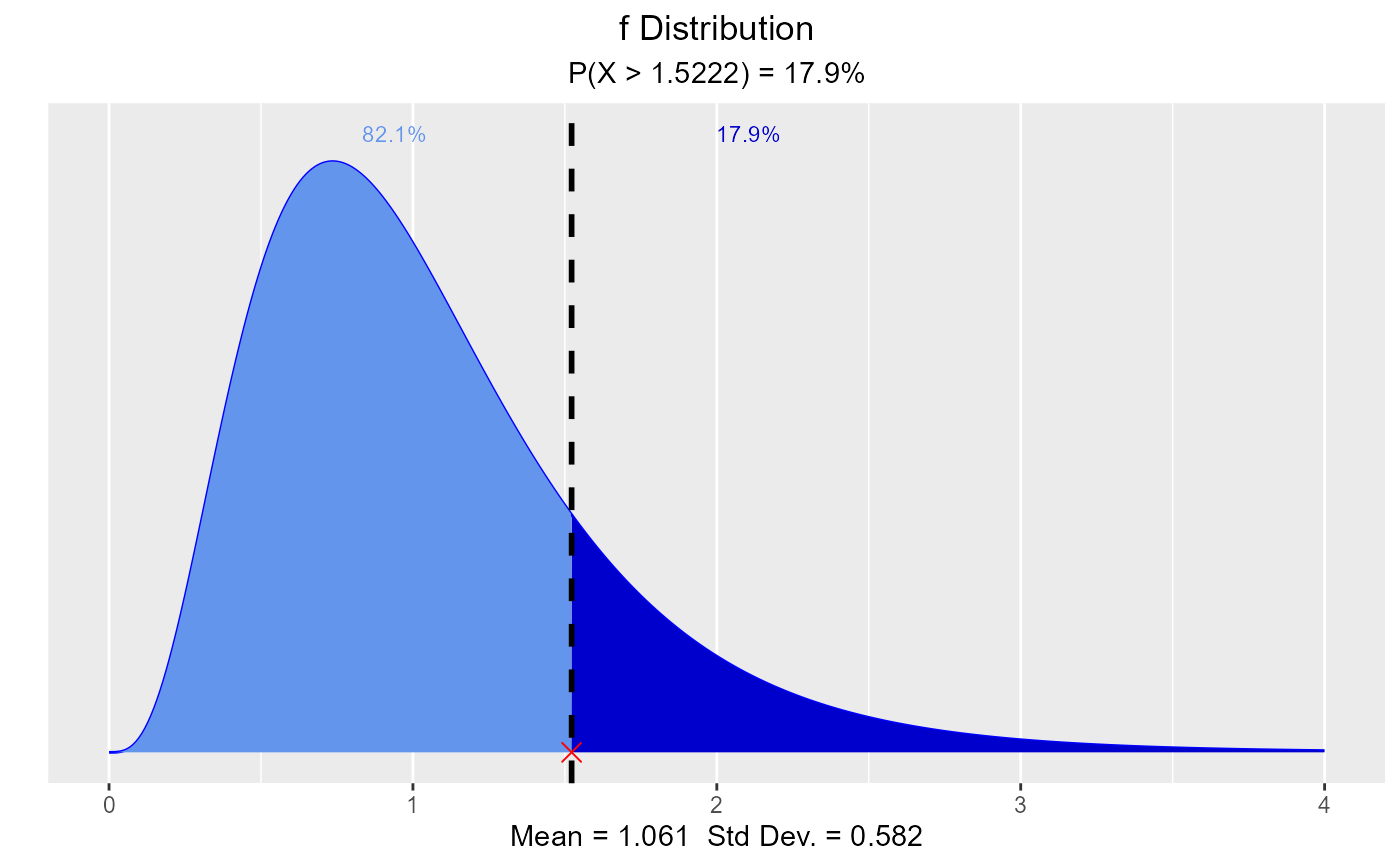Visualize how changes in degrees of freedom affect the shape of the F distribution. Compute & visualize quantiles out of given probability and probability from a given quantile.

vdist_f_plot(num_df = 4, den_df = 30, normal = FALSE, print_plot = TRUE)

vdist_f_perc(
probs = 0.95,
num_df = 3,
den_df = 30,
type = c("lower", "upper"),
print_plot = TRUE
)

vdist_f_prob(
perc = 2.35,
num_df = 5,
den_df = 32,
type = c("lower", "upper"),
print_plot = TRUE
)

## Arguments

num_df Degrees of freedom associated with the numerator of f statistic. Degrees of freedom associated with the denominator of f statistic. If TRUE, normal curve with same mean and sd as the F distribution is drawn. logical; if TRUE, prints the plot else returns a plot object. Probability value. Lower tail or upper tail. Quantile value.

FDist

## Examples

# visualize F distribution
vdist_f_plot()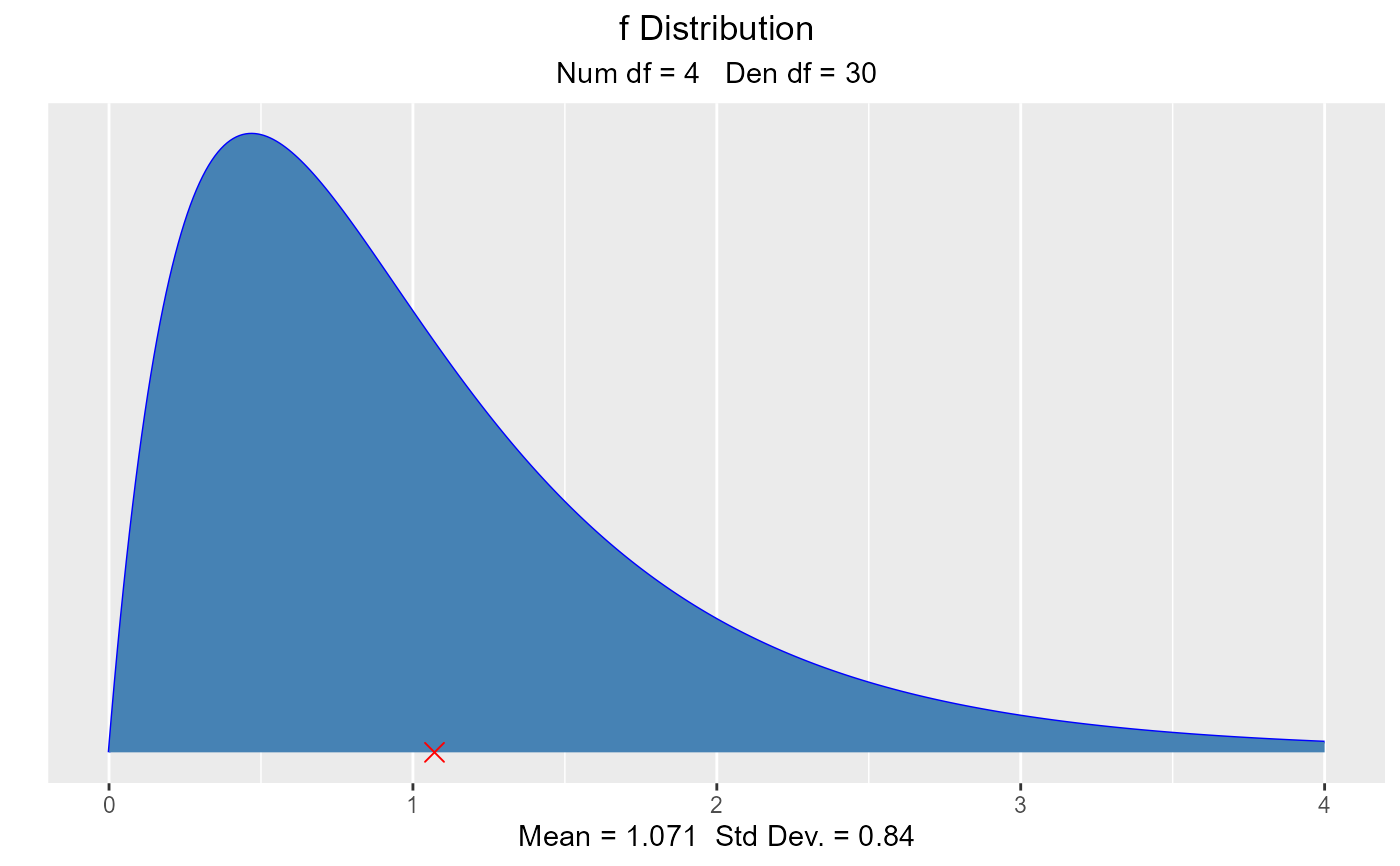vdist_f_plot(6, 10, normal = TRUE)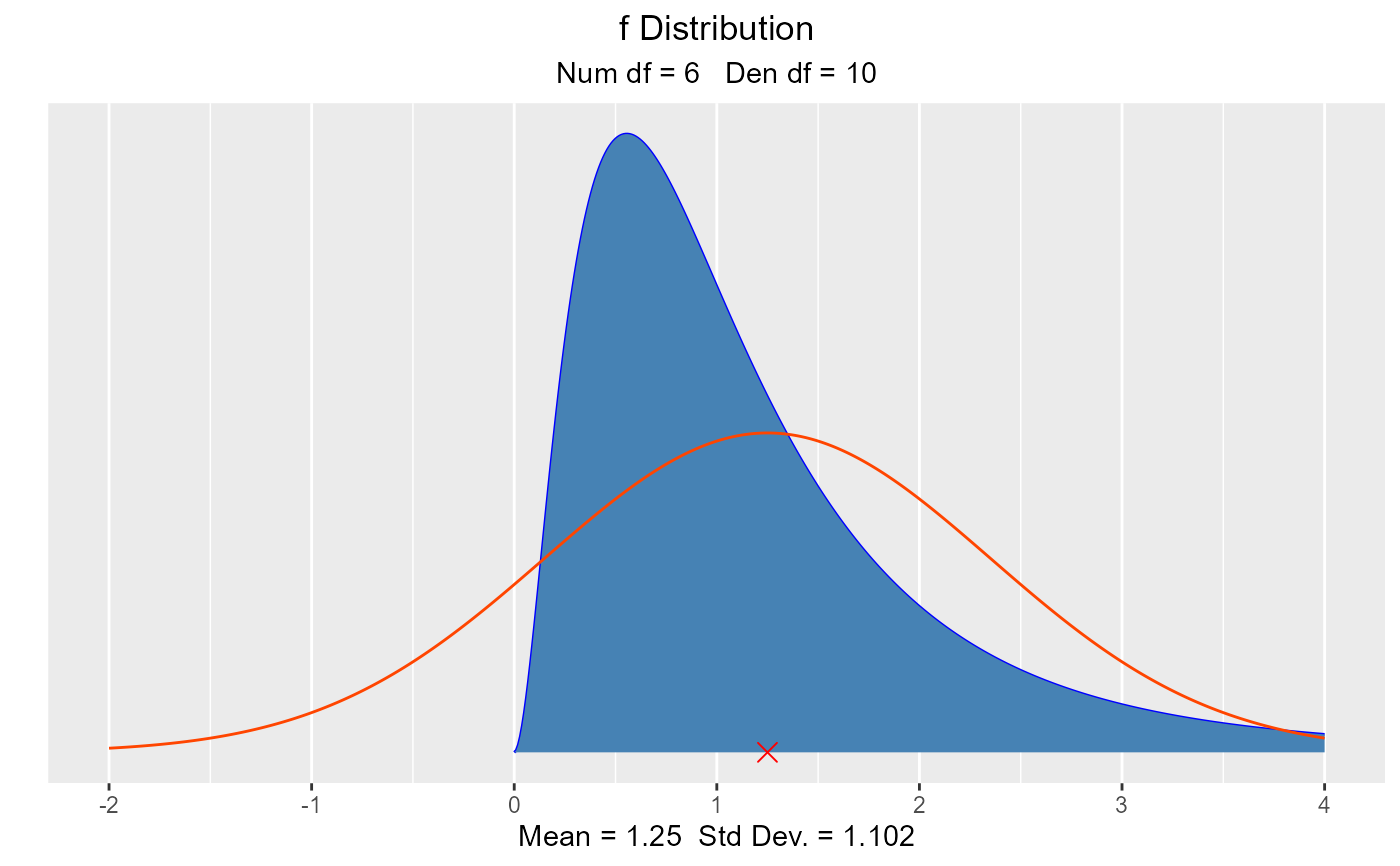# visualize probability from a given quantile
vdist_f_perc(0.95, 3, 30, 'lower')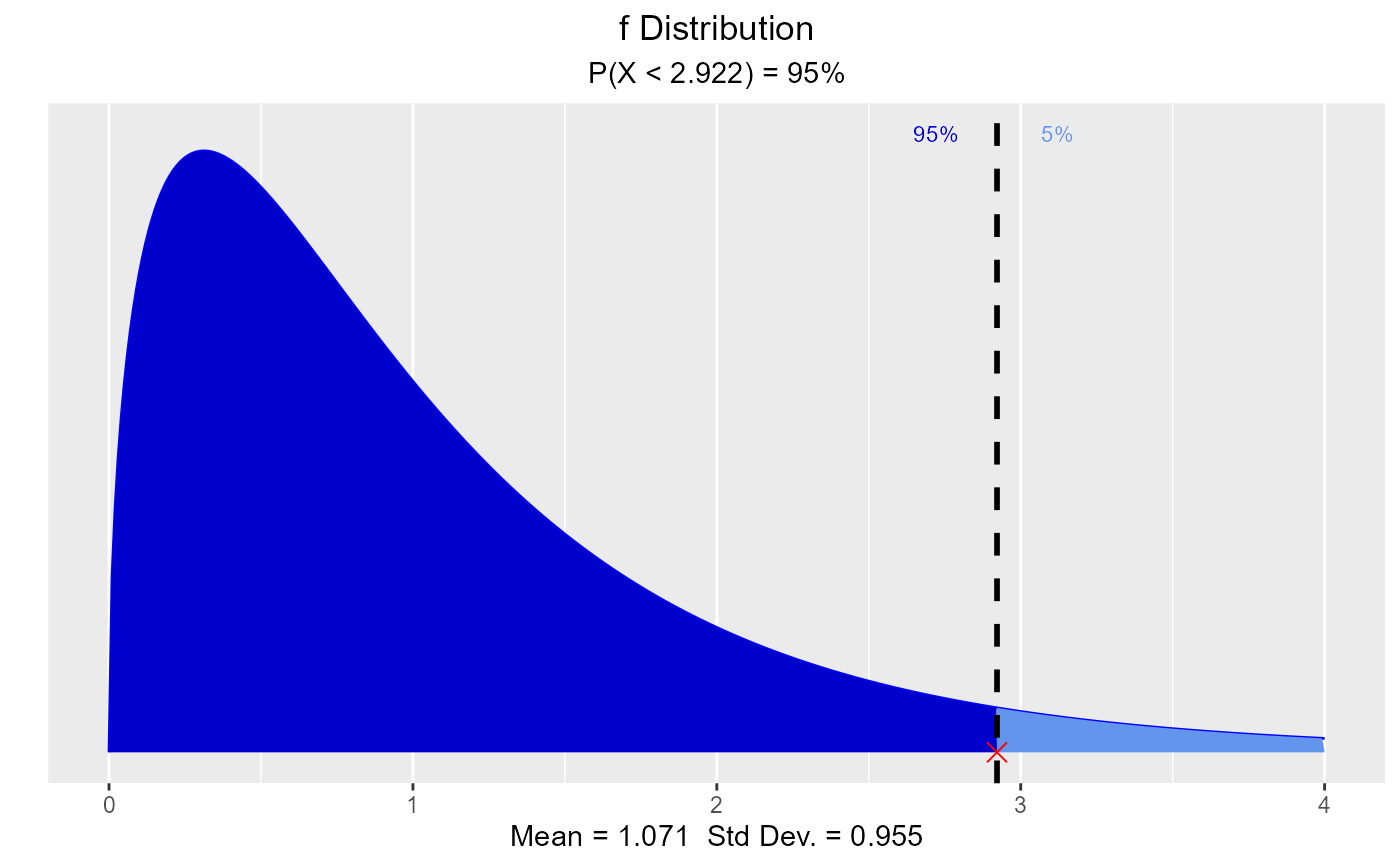vdist_f_perc(0.125, 9, 35, 'upper')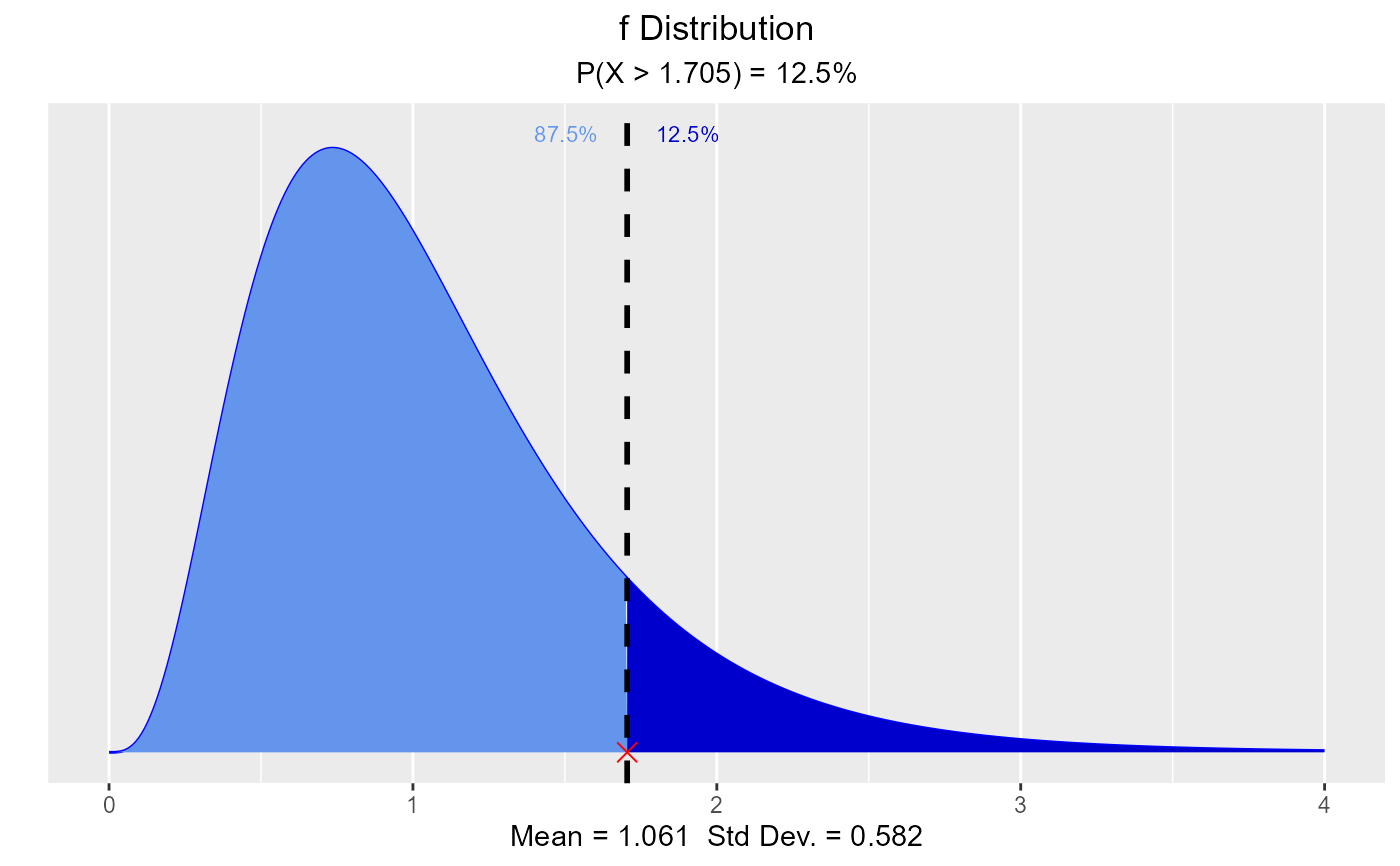# visualize quantiles out of given probability
vdist_f_prob(2.35, 5, 32)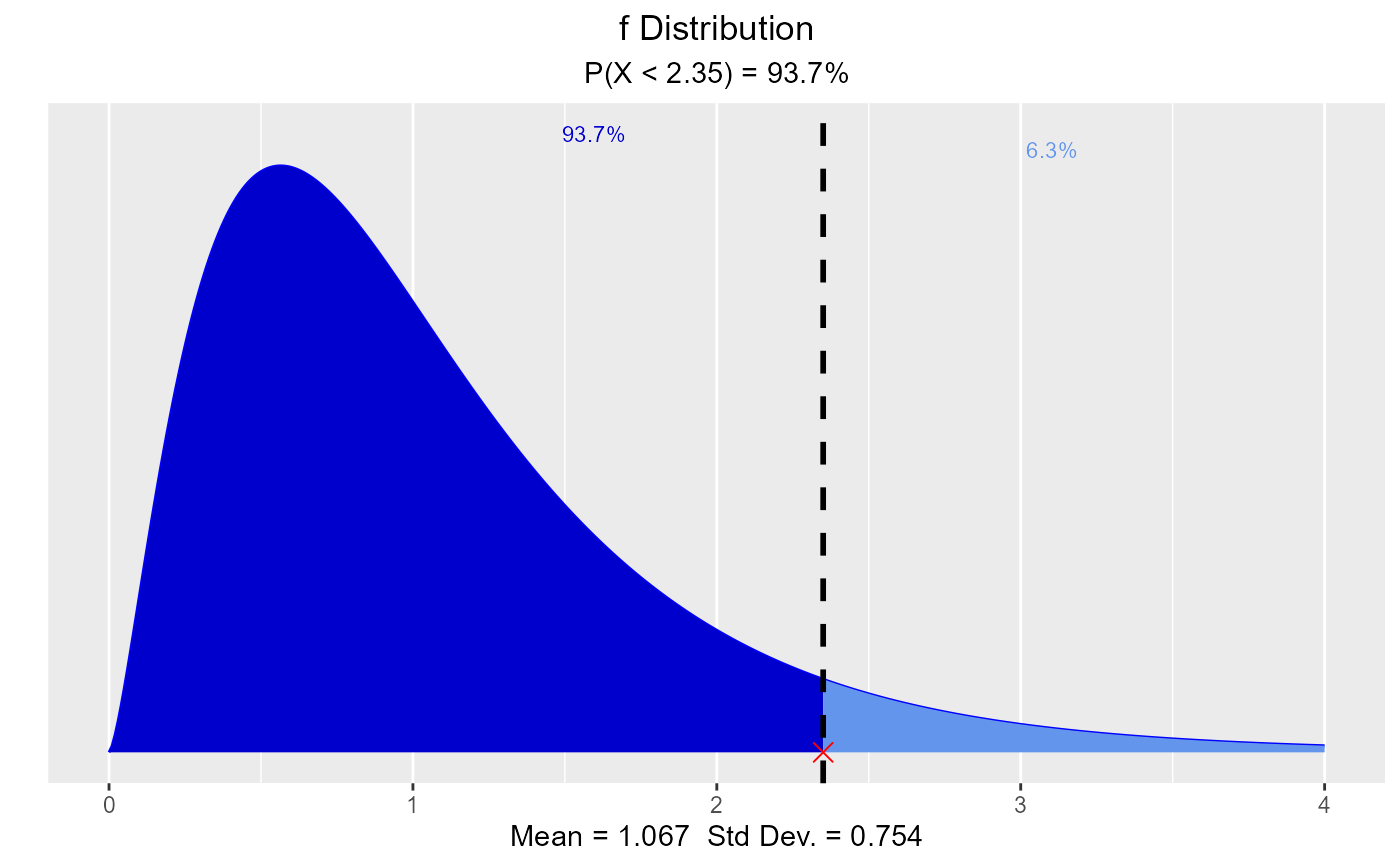vdist_f_prob(1.5222, 9, 35, type = "upper")